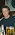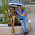## giovedì 19 aprile 2007

### Highlight overlapping area between two curves

This post was updated thanks to Blaž Triglav contribution.
```p <- seq(0.2,1.4,0.01) x1 <- dnorm(p, 0.70, 0.12) x2 <- dnorm(p, 0.90, 0.12)  plot(range(p), range(x1,x2), type = "n")  lines(p, x1, col = "red", lwd = 4, lty = 2)  lines(p, x2, col = "blue", lwd = 4) polygon(c(p,p), c(pmin(x1,x2),0), col = "grey")```
`Below an advanced example (Thanks to Blaž):`
``````p <- seq(54,71,0.1)
x1 <- dnorm(p, 60, 1.5)
x2 <- dnorm(p, 65, 1.5)
par(mar=c(5.5, 4, 0.5, 0.5))
plot(range(p), range(x1,x2), type = "n", xlab="", ylab="", axes=FALSE)
box()
mtext(expression(bar(y)), side=1, line=1, adj=1.0, cex=2, col="black")
mtext("f"~(bar(y)), at=0.265, side=2, line=0.5, adj=1.0, las=2.0, cex=2, col="black")
axis(1, at=60, lab=mu[H]~"=60", cex.axis=1.5, pos=-0.0165, tck=0.02)
axis(1, at=65, lab=mu[H]~"=65", cex.axis=1.5, pos=-0.0165, tck=0.02)
axis(1, at=63.489, lab=y[k][","][zg]~"=63,489", pos=-0.035, tck=0.2)
axis(1, at=63.489, lab="", pos=-0.035, tck=-0.02)
lines(p, x1, col = "black")
lines(p, x2, col = "black")
line3 <- 63.5
polygon(c(line3, p[p<=line3], line3), c(0,x2[p<=line3],0), col = "grey23")
lines(p, x1, col = "black")
line <- 63.489
line1 <- 60
line2 <- 65
abline(v=line, col="black", lwd=1.5, lty = "dashed")
abline(v=line1, col="black", lwd=0.3, lty = "dashed")
abline(v=line2, col="black", lwd=0.3, lty = "dashed")
xt3 <- c(line,p[p>line],line)
yt3 <- c(0,x1[p>line],0)
polygon(xt3, yt3, col="grey")
legend("topright", inset=.05, c(expression(alpha), expression(beta)), fill=c("grey", "grey23"), cex=2)``````

#### 10 commenti:

1.Thank you very much for this post. I am an R novice but this is really helpful for me.

2.I'm glad that my simple tips are of some use for you! An advice: subscribe to the R-help mailing list (http://www.r-project.org/mail.html) it is really a never-ending source of GOOD information, code and inspiration!

3.Will do! Would you mind glancing at my syntax here? I am trying to shade an area under two curves.

#Two curves with shading
p<-seq(-6,9.5,.001)
x1<-dnorm(p,-1.5,1.5)
x2<-dnorm(p,0,1.5)
plot(range(p),range(x1,x2),type="n")
lines(p,x1,col="red",lwd=4)
lines(p,x2,col="blue",lwd=4)
line<- -1.5
abline(v=line)
x<-p
y<-x1
scale<-.001
cutpoint <- (max(x) - line) / scale
xt <- c(x[(length(x)-cutpoint):length(x)],line)
yt <- c(y[(length(y)-cutpoint):length(y)],0)
polygon(xt, yt, density=50)
lines(p,x1,col="red",lwd=4)
lines(p,x2,col="blue",lwd=4)

#As silly as this seems, I can't seem to get the shading to go in the negative direction. Please help, R guru!

4.I'm not at all a guru! I like R because, nine times out of ten, you can solve problems in short time and, of course, because of his awesome community.
I'm not sure if this is what you're looking for...
##
xt1 <- c(x[(length(x)-cutpoint):length(x)], line)
y1=pmin(x1,x2)
yt1 <- c(y1[(length(y1)-cutpoint):length(y1)],0)
polygon(xt1,yt1, density=50)
##

I advice you to take a look at the package HH, the function normal.and.t.dist() may be of particular interest to you!

5.Hey Paolo,
Thanks for getting back to me. Close. I basically used brute force to get to the image I needed although in, I'm sure, a most inefficient manner. I wanted shading on curve x1 from -1.13 to the remainder of the negative tail of the distribution. Maybe you can take a look at at it ans see if you can tell me how one would get this result without using "white" polygon fill to achieve the graphic. You'll see what I'm talking about.
Ryan

p<-seq(-8.5,4.9,.001)
x1<-dnorm(p,-1.5,1.5)
x2<-dnorm(p,0,1.5)
plot(range(p),range(x1,x2),type="n")
lines(p,x1,col="red",lwd=4)
lines(p,x2,col="blue",lwd=4,lty=2)
line<--1.13
#abline(v=line)

x<-p
y<-x1
scale<-.001
shade<- (max(y)) / scale
polygon(xt, yt, col="gray")
lines(p,x1,col="red",lwd=4)
lines(p,x2,col="blue",lwd=4,lty=2)

x<-p
y<-x1
scale<-.001
cutpoint <- (max(x)-line) / scale
xt <- c(x[(length(x)-cutpoint):length(x)],line)
yt <- c(y[(length(y)-cutpoint):length(y)],0)
polygon(xt, yt, col='white')
lines(p,x1,col="red",lwd=4)
lines(p,x2,col="blue",lwd=4,lty=2)
lines(p,x1,col="red",lwd=4)
floor<-0
abline(h=floor)

6.This should fit your need:

p = seq(-8.5,4.9,.001)
x1 <- dnorm(p,-1.5,1.5)
x2 <- dnorm(p,0,1.5)
plot(range(p),range(x1,x2),type="n")
lines(p,x1,col="red",lwd=4)
lines(p,x2,col="blue",lwd=4,lty=2)
line <-- 1.13
abline(v=line)

xt1 <- c(p, p[p < line], line)
yt1 <- c(x1, x1[p < line],0)
polygon(xt1, yt1, col="grey")

7.Paolo, you're a genius! Thank you for all your help and recommendations. I look forward to chatting in the future.

Best wishes,
Ryan

8.You're too kind! :-S
Glad I was useful! Thanks for your contribution! I hope that someone else will find our effort useful.

9.Hello. This is great R code but I cannot use the above code because I transform data (please see below). Is there any way to highlight overlapping area between two curves?

... such like
a1=-0.116977231
b1=0.776585015
c1=0.116977231
d1=0.065499503

y1=rnorm(1000000,0,1)
y1=a1+b1*y1+c1*y1^2+d1*y1^3
y2=rnorm(1000000,0,1)
y2=a1+b1*y2+c1*y2^2+d1*y2^3
y2=2.5*y2+0.5

plot(density(y1), xlim= c(-7, 7), ylim =c(0, 0.75), col="black", lwd=2, lty=1, xlab=" ", main="",bty="l" )
lines(density(y2), xlim= c(-7, 7), ylim =c(0, 0.75), col="black", lwd=2, lty=2, xlab=" ", main="")

Many thanks..

Yoonjeong

1.Dear Yoonjeong,
Not a solution, but a starting point:

library(ggplot2)
ggplot()+ geom_density(aes(x=y1), colour="red", fill="red", alpha=0.3) + geom_density(aes(x=y2), colour="blue", fill="blue", alpha=0.3)

HIH!